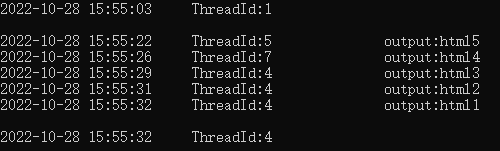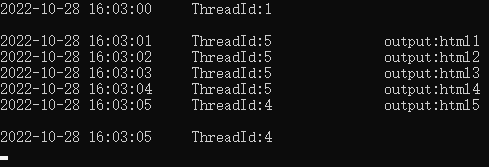# C# 8.0 添加和增强的功能【基础篇】

123 0

`.NET Core 3.x``.NET Standard 2.1`支持`C# 8.0`

``````public struct Point
{
public double X { get; set; }
public double Y { get; set; }
public double Distance => Math.Sqrt(X * X + Y * Y);
public override string ToString() =>
\$"({X}, {Y}) is {Distance} from the origin";
}``````

``````public readonly override string ToString() =>// 编译器警告，因为 ToString 访问未标记为 readonly 的 Distance 属性
\$"({X}, {Y}) is {Distance} from the origin";
public readonly double Distance => Math.Sqrt(X * X + Y * Y);``````

## 二、默认接口方法

`.NET Core 3.0` 上的 `C# 8.0` 开始，可以在声明接口成员时定义实现。 最常见的方案是，可以将成员添加到已经由无数客户端发布并使用的接口。示例：

``````// 先声明两个接口
// 客户接口
public interface ICustomer
{
IEnumerable<IOrder> PreviousOrders { get; }
DateTime DateJoined { get; }
DateTime? LastOrder { get; }
string Name { get; }
IDictionary<DateTime, string> Reminders { get; }

// 在客户接口中加入新的方法实现
public decimal ComputeLoyaltyDiscount()
{
if ((DateJoined < TwoYearsAgo) && (PreviousOrders.Count() > 10))
{
return 0.10m;
}
return 0;
}
}
// 订单接口
public interface IOrder
{
DateTime Purchased { get; }
decimal Cost { get; }
}

// 测试代码
// SampleCustomer：接口 ICustomer 的实现，可不实现方法 ComputeLoyaltyDiscount
// SampleOrder：接口 IOrder 的实现
SampleCustomer c = new SampleCustomer("customer one", new DateTime(2010, 5, 31))
{
Reminders =
{
{ new DateTime(2010, 08, 12), "childs's birthday" },
{ new DateTime(1012, 11, 15), "anniversary" }
}
};

SampleOrder o = new SampleOrder(new DateTime(2012, 6, 1), 5m);
o = new SampleOrder(new DateTime(2103, 7, 4), 25m);

// 验证新增的接口方法
ICustomer theCustomer = c; // 从 SampleCustomer 到 ICustomer 的强制转换
Console.WriteLine(\$"Current discount: {theCustomer.ComputeLoyaltyDiscount()}");
// 若要调用在接口中声明和实现的任何方法，该变量的类型必须是接口类型，即：theCustomer``````

## 三、模式匹配的增强功能

`C# 8.0`扩展了`C# 7.0`中的词汇表（`is``switch`），这样就可以在代码中的更多位置使用更多模式表达式。

## 3.1 switch 表达式

变量位于 switch 关键字之前；

将 case 和 : 元素替换为 =>，更简洁、直观；

将 default 事例替换为 _ 弃元；

实际语句是表达式，比语句更加简洁。

``````public static RGBColor FromRainbow(Rainbow colorBand) =>
colorBand switch
{
Rainbow.Red    => new RGBColor(0xFF, 0x00, 0x00),
Rainbow.Orange => new RGBColor(0xFF, 0x7F, 0x00),
Rainbow.Yellow => new RGBColor(0xFF, 0xFF, 0x00),
Rainbow.Green  => new RGBColor(0x00, 0xFF, 0x00),
Rainbow.Blue   => new RGBColor(0x00, 0x00, 0xFF),
Rainbow.Indigo => new RGBColor(0x4B, 0x00, 0x82),
Rainbow.Violet => new RGBColor(0x94, 0x00, 0xD3),
_              => throw new ArgumentException(message: "invalid enum value", paramName: nameof(colorBand)),
};``````

## 3.2 属性模式

``````// Address：地址对象；salePrice：售价
public static decimal ComputeSalesTax(Address location, decimal salePrice) =>
location switch
{
{ State: "WA" } => salePrice * 0.06M,
{ State: "MN" } => salePrice * 0.075M,
{ State: "MI" } => salePrice * 0.05M,
// other cases removed for brevity...
_ => 0M
};``````

## 3.3 元组模式

``````// 游戏“rock, paper, scissors（石头剪刀布）”的切换表达式
public static string RockPaperScissors(string first, string second)
=> (first, second) switch
{
("rock", "paper") => "rock is covered by paper. Paper wins.",
("rock", "scissors") => "rock breaks scissors. Rock wins.",
("paper", "rock") => "paper covers rock. Paper wins.",
("paper", "scissors") => "paper is cut by scissors. Scissors wins.",
("scissors", "rock") => "scissors is broken by rock. Rock wins.",
("scissors", "paper") => "scissors cuts paper. Scissors wins.",
(_, _) => "tie" // 此处弃元 表示平局(石头剪刀布游戏)的三种组合或其他文本输入
};``````

## 3.4 位置模式

``````// 位于象限中的 点对象
public class Point
{
public int X { get; }
public int Y { get; }
public Point(int x, int y) => (X, Y) = (x, y);
public void Deconstruct(out int x, out int y) => (x, y) = (X, Y);
}
{
Unknown, Origin, One, Two, Three, Four, OnBorder
}
// 下面的方法使用位置模式来提取 x 和 y 的值。 然后，它使用 when 子句来确定该点的 Quadrant
{
var (x, y) when x > 0 && y > 0 => Quadrant.One,
var (x, y) when x < 0 && y > 0 => Quadrant.Two,
var (x, y) when x < 0 && y < 0 => Quadrant.Three,
var (x, y) when x > 0 && y < 0 => Quadrant.Four,
var (_, _) => Quadrant.OnBorder,// 当 x 或 y 为 0（但不是两者同时为 0）时，前一个开关中的弃元模式匹配
};``````

## 四、using 声明

using 声明是前面带 `using` 关键字的变量声明。它指示编译器声明的变量应在封闭范围的末尾进行处理。

``````static int WriteLinesToFile(IEnumerable<string> lines)
{
using var file = new System.IO.StreamWriter("WriteLines2.txt");
int skippedLines = 0;
foreach (string line in lines)
{
if (!line.Contains("Second"))
file.WriteLine(line);
else
skippedLines++;
}
return skippedLines;
// 当代码运行到此位置时，file 被销毁
// 相当于 using (var file = new System.IO.StreamWriter("WriteLines2.txt")){ ... }
}``````

## 五、静态本地函数

`C# 8.0`中可以向本地函数添加 `static` 修饰符，以确保本地函数不会从封闭范围捕获（引用）任何变量。 若引用了就会生成报错：`CS8421`-“静态本地函数不能包含对 <variable> 的引用”。

``````// 本地方法 LocalFunction 访问了方法 M() 这个封闭空间的变量 y
// 因此，不能用 static 修饰符来声明
int M()
{
int y;
LocalFunction();
return y;
void LocalFunction() => y = 0;
}``````
``````// Add 方法可以是静态的，因为它不访问封闭范围内的任何变量
int M()
{
int y = 5;
int x = 7;
static int Add(int left, int right) => left + right;
}``````

## 六、可处置的 ref 结构

``````class Program
{
static void Main(string[] args)
{
using (var book = new Book())
Console.WriteLine("Hello World!");
}
}
// 错误写法
// Error CS8343 'Book': ref structs cannot implement interfaces
ref struct Book : IDisposable
{
public void Dispose()
{   }
}
// 正确写法
class Program
{
static void Main(string[] args)
{
// 根据 using 新特性，简洁的写法，默认在当前代码块结束前销毁对象 book
using var book = new Book();
// ...
}
}
ref struct Book
{
public void Dispose()
{
}
}``````

## 八、异步流

``````// 异步枚举，核心对象是：IAsyncEnumerable
[HttpGet("syncsale")]
public async IAsyncEnumerable<Product> GetOnSaleProducts()
{
var products = _repository.GetProducts();
await foreach (var product in products) // 消费异步枚举，顺序取决于 IAsyncEnumerator 算法
{
if (product.IsOnSale)
yield return product;// 持续异步逐个返回，不用等全部完成
}
}``````

``````// 这是一个【相互独立的长耗时行为的集合（假设分别耗时 5,4,3,2,1s）】
{
await foreach (var html in FetchAllHtml()) // 默认按照任务加入的顺序输出
{
}
}
// 这里已经默认实现了一个 IEnumerator 枚举器： 以 for 循环加入异步任务的顺序
static async IAsyncEnumerable<string> FetchAllHtml()
{
for (int i = 5; i >= 1; i--)
{
var html = await Task.Delay(i* 1000).ContinueWith((t,i)=> \$"html{i}",i); // 模拟长耗时
yield return html;
}
}````````````static async IAsyncEnumerable<string> FetchAllHtml()
{
for (int i = 5; i >= 1; i--)
{
}
{
yield return await tFinlish; // 完成即输出
}
}  ``````## 九、异步可释放（IAsyncDisposable）

`IAsyncDisposable `接口，提供一种用于异步释放非托管资源的机制。与之对应的就是提供同步释放非托管资源机制的接口 `IDisposable`

``````public class ExampleClass : IAsyncDisposable
{
private Stream _memoryStream = new MemoryStream();
public ExampleClass()
{	}
{
await _memoryStream.DisposeAsync();
}
}
// using 语法糖
await using var s = new ExampleClass()
{
// doing
};
// 优化 同样是对象 s 只存在于当前代码块
await using var s = new ExampleClass();
// doing``````

## 十、索引和范围

``````var words = new string[]
{
// index from start    index from end
"The",      // 0                   ^9
"quick",    // 1                   ^8
"brown",    // 2                   ^7
"fox",      // 3                   ^6
"jumped",   // 4                   ^5

"over",     // 5                   ^4
"the",      // 6                   ^3
"lazy",     // 7                   ^2
"dog"       // 8                   ^1

};              // 9 (or words.Length) ^0``````

``````Console.WriteLine(\$"The last word is {words[^1]}");
// “dog” // 使用 ^1 索引检索最后一个词

var quickBrownFox = words[1..4];
//“quick”、“brown”、“fox” 子范围

var lazyDog = words[^2..^0];
// “lazy”、“dog” 子范围

var allWords = words[..];
// “The”、“dog”子范围
var firstPhrase = words[..4];
// “The”、“fox”子范围
var lastPhrase = words[6..];
// “the”、“lazy”、“dog”子范围``````

``````Range phrase = 1..4;
var text = words[phrase];``````

## 十一、 Null 合并赋值

Null 合并赋值运算符：`??=`

``````List<int> numbers = null;
int? i = null;
numbers ??= new List<int>();
Console.WriteLine(string.Join(" ", numbers));  // output: 17 17
Console.WriteLine(i);  // output: 17``````

## 十二、非托管构造类型

``````public struct Coords<T>
{
public T X;
public T Y;
}
// Coords<int> 类型为 C# 8.0 及更高版本中的非托管类型
// 与任何非托管类型一样，可以创建指向此类型的变量的指针，或针对此类型的实例在堆栈上分配内存块
Span<Coords<int>> coordinates = stackalloc[]
{
new Coords<int> { X = 0, Y = 0 },
new Coords<int> { X = 0, Y = 3 },
new Coords<int> { X = 4, Y = 0 }
};``````

Span 简介

在定义中，Span 就是一个简单的值类型。它真正的价值，在于允许我们与任何类型的连续内存一起工作。

在使用中，Span 确保了内存和数据安全，而且几乎没有开销。

要使用 Span，需要设置开发语言为 C# 7.2 以上，并引用`System.Memory`到项目。

Span 使用时，最简单的，可以把它想象成一个数组，有一个`Length`属性和一个允许读写的`index`

``````// 常用的一些定义、属性和方法
Span(T[] array);
Span(T[] array, int startIndex);
Span(T[] array, int startIndex, int length);
unsafe Span(void* memory, int length);
int Length { get; }
ref T this[int index] { get; set; }
Span<T> Slice(int start);
Span<T> Slice(int start, int length);
void Clear();
void Fill(T value);
void CopyTo(Span<T> destination);
bool TryCopyTo(Span<T> destination);
// 从 T[] 到 Span 的隐式转换
char[] array = new char[] { 'i', 'm', 'p', 'l', 'i', 'c', 'i', 't' };
Span<char> fromArray = array;
// 复制内存

Span 参考： 关于C# Span的一些实践

## 十三、嵌套表达式中的 stackalloc

``````Span<int> numbers = stackalloc[] { 1, 2, 3, 4, 5, 6 };
var ind = numbers.IndexOfAny(stackalloc[] { 2, 4, 6, 8 });
Console.WriteLine(ind);  // output: 1``````

stackalloc 表达式简介：

stackalloc 关键字用于不安全的代码上下文中，以便在堆栈上分配内存块。

``````// 关键字仅在局部变量的初始值中有效，正确写法：
int* block = stackalloc int;
// 错误写法：
int* block;
block = stackalloc int;``````

由于涉及指针类型，因此 stackalloc 要求不安全上下文。

以下代码示例计算并演示 Fibonacci 序列中的前 20 个数字。 每个数字是先前两个数字的和。 在代码中，大小足够容纳 20 个 int 类型元素的内存块是在堆栈上分配的，而不是在堆上分配的。  该块的地址存储在 fib 指针中。 此内存不受垃圾回收的制约，因此不必将其钉住（通过使用 fixed）。 内存块的生存期受限于定义它的方法的生存期。 不能在方法返回之前释放内存。

``````class Test
{
static unsafe void Main()
{
const int arraySize = 20;
int* fib = stackalloc int[arraySize];
int* p = fib;
*p++ = *p++ = 1;// The sequence begins with 1, 1.
for (int i = 2; i < arraySize; ++i, ++p)
*p = p[-1] + p[-2];// Sum the previous two numbers.
for (int i = 0; i < arraySize; ++i)
Console.WriteLine(fib[i]);
// Keep the console window open in debug mode.
System.Console.WriteLine("Press any key to exit.");
}
}
/*
Output
1
1
2
3
5
8
13
21
34
55
89
144
233
377
610
987
1597
2584
4181
6765
*/``````

不安全代码的安全性低于安全替代代码。 但是，通过使用 stackalloc 可以自动启用公共语言运行时 (CLR) 中的缓冲区溢出检测功能。 如果检测到缓冲区溢出，进程将尽快终止，以最大限度地减小执行恶意代码的机会。

stackalloc 表达式参考：C#不安全代码和stackalloc

## 十四、内插逐字字符串的增强功能

• 这篇文章还没有收到评论，赶紧来抢沙发吧~